+

# Introduction to Order of Operations

##### Rating:
(1)
• (0)
• (1)
• (0)
• (0)
• (0)
Author: Sophia Tutorial
##### Description:

Evaluate an expression using Order of Operations

(more)
Tutorial
what's covered
1. Introduction to Order of Operations

# 1. Introduction to Order of Operations

When simplifying expressions, it is important that we simplify them in the correct order. Consider the following problem done two different ways: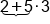Add First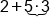Multiply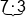Multiply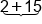Add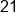Solution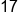Solution

The previous example illustrates that if the same problem is done two different ways we will arrive at two different solutions. However, only one method can be correct. It turns out the second method, 17, is the correct method. The order of operations ends with the most basic of operations, addition (or subtraction). Before addition is completed we must do repeated addition or multiplication (or division). Before multiplication is completed we must do repeated multiplication or exponents. When we want to do something out of order and make it come first we will put it in parenthesis (or grouping symbols). This list then is our order of operations we will use to simplify expressions.

big idea
Order of Operations: Parentheses (grouping), Exponents (radicals), Multiply & Divide (left to right), Add & Subtract (left to right) Multiply and Divide are on the same level because they are the same operation (division is just multiplying by the reciprocal). This means they must be done left to right, so some problems we will divide first, others we will multiply first. The same is true for adding and subtracting (subtracting is just adding the opposite).

hint
Often students use the word PEMDAS to remember the order of operations, as the first letter of each operation creates the word PEMDAS. However, it is useful to think about PEMDAS as a vertical word written as: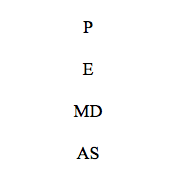term to know
PEMDAS
An acronym to remember the order of operations: parentheses, exponents, multiplication and division, addition and subtraction.

This format helps us remember that multiplication and division are done left to right (same with addition and subtraction). Another way students remember the order of operations is to think of a phrase such as “Please Excuse My Dear Aunt Sally” where each word starts with the same letters as the order of operations start with.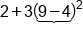Parenthesis first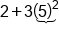Exponents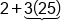Multiply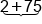Add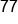Our Solution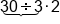Divide first (left to right!)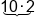Multiply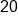Our Solution

In the previous example, if we had multiplied first, five would have been the answer which is incorrect. If there are several parenthesis in a problem we will start with the inner most parenthesis and work our way out. Inside each parenthesis we simplify using the order of operations as well. To make it easier to know which parenthesis goes with which parenthesis, different types of parenthesis will be used such as { } and [] and ( ), these parenthesis all mean the same thing, they are parenthesis and must be evaluated first.

summary
As the above example illustrates, it can take several steps to complete a problem. With order of operations, we can use the acronym PEMDAS, which stands for Parentheses (grouping), Exponents (radicals), Multiply & Divide (left to right), Add & Subtract (left to right). Remember that multiplication and division are actually done together moving from left to right. Addition and subtraction are also done together, just moving from left to right. The key to successfully solve order of operations problems is to take the time to show your work and do one step at a time. This will reduce the chance of making a mistake along the way.

Source: Adapted from "Beginning and Intermediate Algebra" by Tyler Wallace, an open source textbook available at: http://wallace.ccfaculty.org/book/book.html

Terms to Know
PEMDAS

An acronym to remember the order of operations: parentheses, exponents, multiplication and division, addition and subtraction.

Rating Header